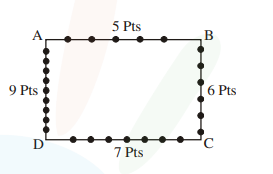# Consider a rectangle ABCD having 5,7,6,9 points

Question:

Consider a rectangle ABCD having $5,7,6,9$ points in the interior of the line segments $\mathrm{AB}$, $\mathrm{CD}, \mathrm{BC}, \mathrm{DA}$

respectively. Let $\alpha$ be the number of triangles having these points from different sides as vertices and $\beta$ be the number of quadrilaterals

having these points from different sides as vertices. Then $(\beta-\alpha)$ is equal to :

1. 795

2. 1173

3. 1890

4. 717

Correct Option: , 4

Solution:$\alpha=$ Number of triangles

$\alpha=5 \cdot 6 \cdot 7+5 \cdot 7 \cdot 9+5 \cdot 6 \cdot 9+6 \cdot 7 \cdot 9$

$=210+315+270+378$

$=1173$

$\beta=$ Number of Quadrilateral

$\beta=5 \cdot 6 \cdot 7 \cdot 9=1890$

$\beta-\alpha=1890-1173=717$